Next: von Neumann stability analysis Up: The diffusion equation Previous: An example 1-d diffusion

## An example 1-d solution of the diffusion equation

Let us now solve the diffusion equation in 1-d using the finite difference technique discussed above. We seek the solution of Eq. (191) in the region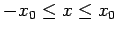, subject to the initial condition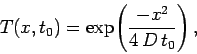(200)

where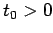. The spatial boundary conditions are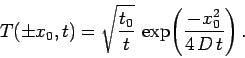(201)

Of course, we can solve this problem analytically to give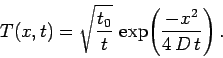(202)

Note that the above equation describes a Gaussian pulse which gradually decreases in height and broadens in width in such a manner that its area is conserved. The width of the pulse varies approximately as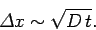(203)

Moreover, the pulse approaches a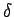-function as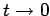.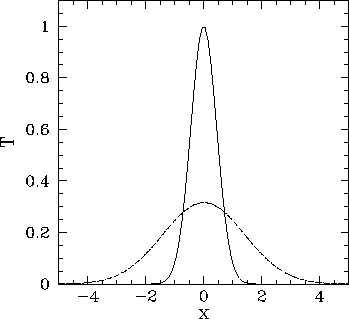Figure 71 shows a comparison between the analytic and numerical solutions for a calculation performed using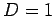,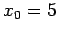,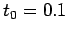,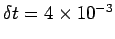, and. It can be seen that the analytic and numerical solutions are in excellent agreement.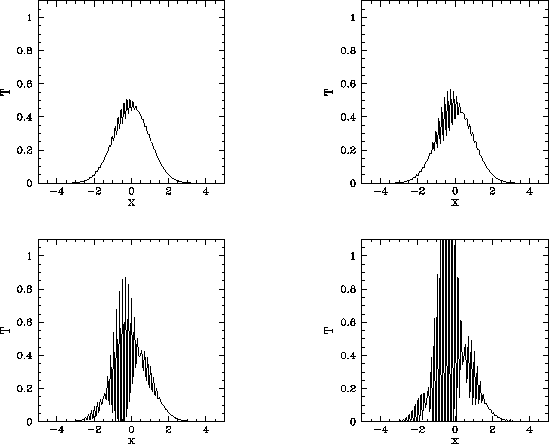It is reasonable to expect that asincreases at fixed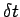(i.e., the spatial resolution increases at fixed temporal resolution) the numerical solution should become more and more accurate. This is indeed the case--at least, untilexceeds a critical value. Beyond this value, there is a catastrophic breakdown in the numerical solution. This breakdown is illustrated in Fig. 72. It can be seen that the solution develops rapidly growing short-wavelength oscillations. Indeed, the solution eventually becomes effectively infinite. Let us investigate this unusual and rather disturbing phenomenon.Next: von Neumann stability analysis Up: The diffusion equation Previous: An example 1-d diffusion
Richard Fitzpatrick 2006-03-29